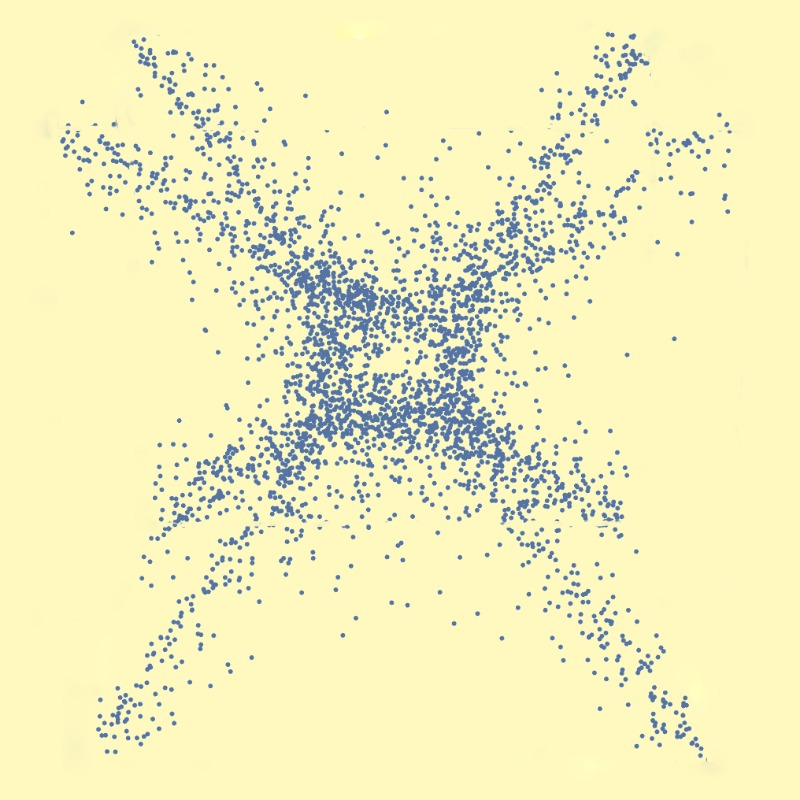# Mahler measuringamoebae

Algebraic geometry

Genetic symbolic regression methods reveal the relationship between amoebae from tropical geometry and the Mahler measure from number theory.

## Mahler measuring the genetic code of amoebae

In Press Advances in Theoretical and Mathematical Physics (2023)

S. Chen, Y. He, E. Hirst, A. Nestor, A. Zahabi

Amoebae from tropical geometry and the Mahler measure from number theory play important roles in quiver gauge theories and dimer models. Their dependencies on the coefficients of the Newton polynomial closely resemble each other, and they are connected via the Ronkin function. Genetic symbolic regression methods are employed to extract the numerical relationships between the 2d and 3d amoebae components and the Mahler measure. We find that the volume of the bounded complement of a d-dimensional amoeba is related to the gas phase contribution to the Mahler measure by a degree-d polynomial, with d = 2 and 3. These methods are then further extended to numerical analyses of the non-reflexive Mahler measure. Furthermore, machine learning methods are used to directly learn the topology of 3d amoebae, with strong performance. Additionally, analytic expressions for boundaries of certain amoebae are given.

In Press Advances in Theoretical and Mathematical Physics (2023)

S. Chen, Y. He, E. Hirst, A. Nestor, A. Zahabi